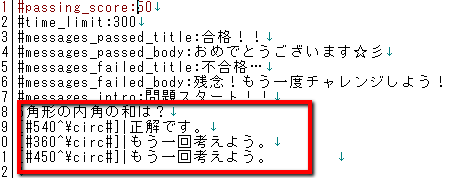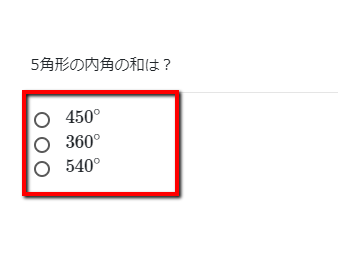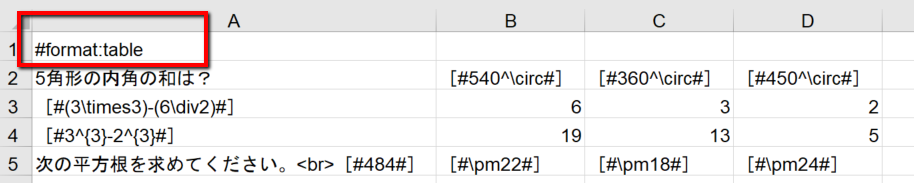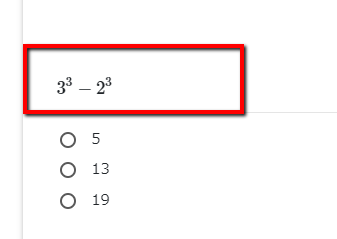• HOME
• Let's create some math problems!
Do you think it is difficult to create a mathematical problem?
Using the quiz generator, once you learn how to make it, you can
I can easily create math problems in text, spreadsheet software!!!
Now let's actually create a formula problem~♪

## How to make formula problems

In Latex formatnumerical formulaBy writing thenumerical formulaSetup usingask a questionhandIt is easy to create.
[# and #] in the LaTeX format betweennumerical formulaby writingIn the image.It will be converted and displayed in the question.
You can also use it within the commentary.

So.List of LaTeX command sheetsPlease see the following.

You can also see an example of inputting a mathematical expression in LaTeX format at the following site.

The following sites arehanddocumentfoliagea number ofequationand automatically recognizes LaTeX and MathThis is a service to convert and output to ML.
Please make use of it if you like.
~ Formula handwriting site ~

## Example of creating a problem

### When created with textThe LaTex type formula you created above will appear as follows!You can check the problems you have created here! →→→Mathematical Problems ★★★

### When created with spreadsheet softwareWhen you create it by spreadsheet software, write "#format:table" in column A of the first matrix.

Fill in the question text in column A below, and write the correct and incorrect answers in columns B, C, and D.

If you write the equation in the same way as the text, the

The problem in column 4 appears as follows!You can also set the following options for the quiz
How to set the options → → → → list of options

I urge you to try the quiz generator to see if you can actually solve a math problem!